# Selina Solutions Concise Mathematics Class 6 Chapter 15: Decimal Fractions Exercise 15(A)

Selina Solutions Concise Mathematics Class 6 Chapter 15: Decimal Fractions Exercise 15(A) explains concepts like conversion of decimal fractions into fractions and vice versa and decimal places. The solutions prepared by the faculty at BYJU’S in PDF format, help students ace the exam with high marks. Frequent practice of exercise wise problems helps them to increase analytical thinking, which is significant from an exam point of view. Students can download the Selina Solutions Concise Mathematics Class 6 Chapter 15 Decimal Fractions Exercise 15(A) free PDF, from the links available below.

## Selina Solutions Concise Mathematics Class 6 Chapter 15: Decimal Fractions Exercise 15(A) Download PDF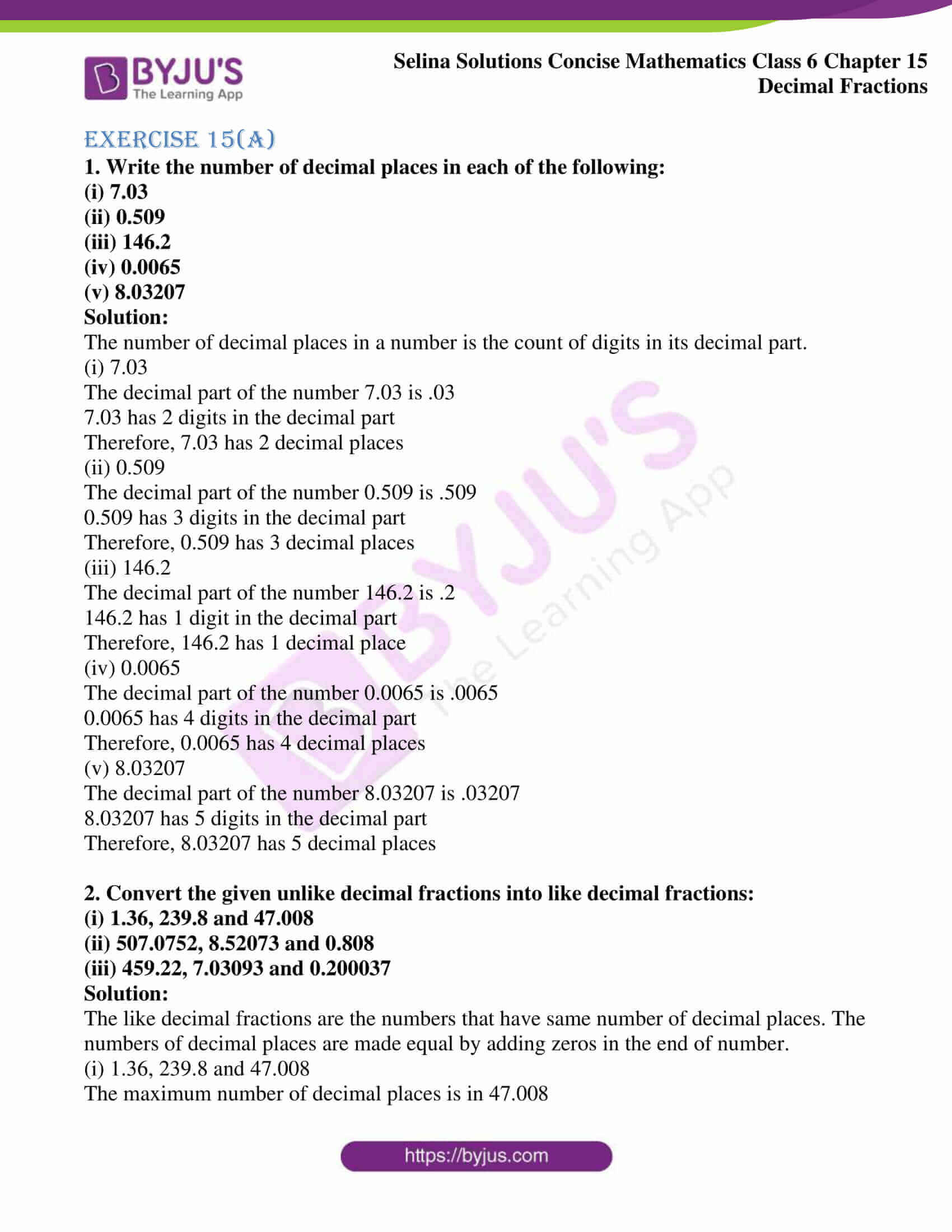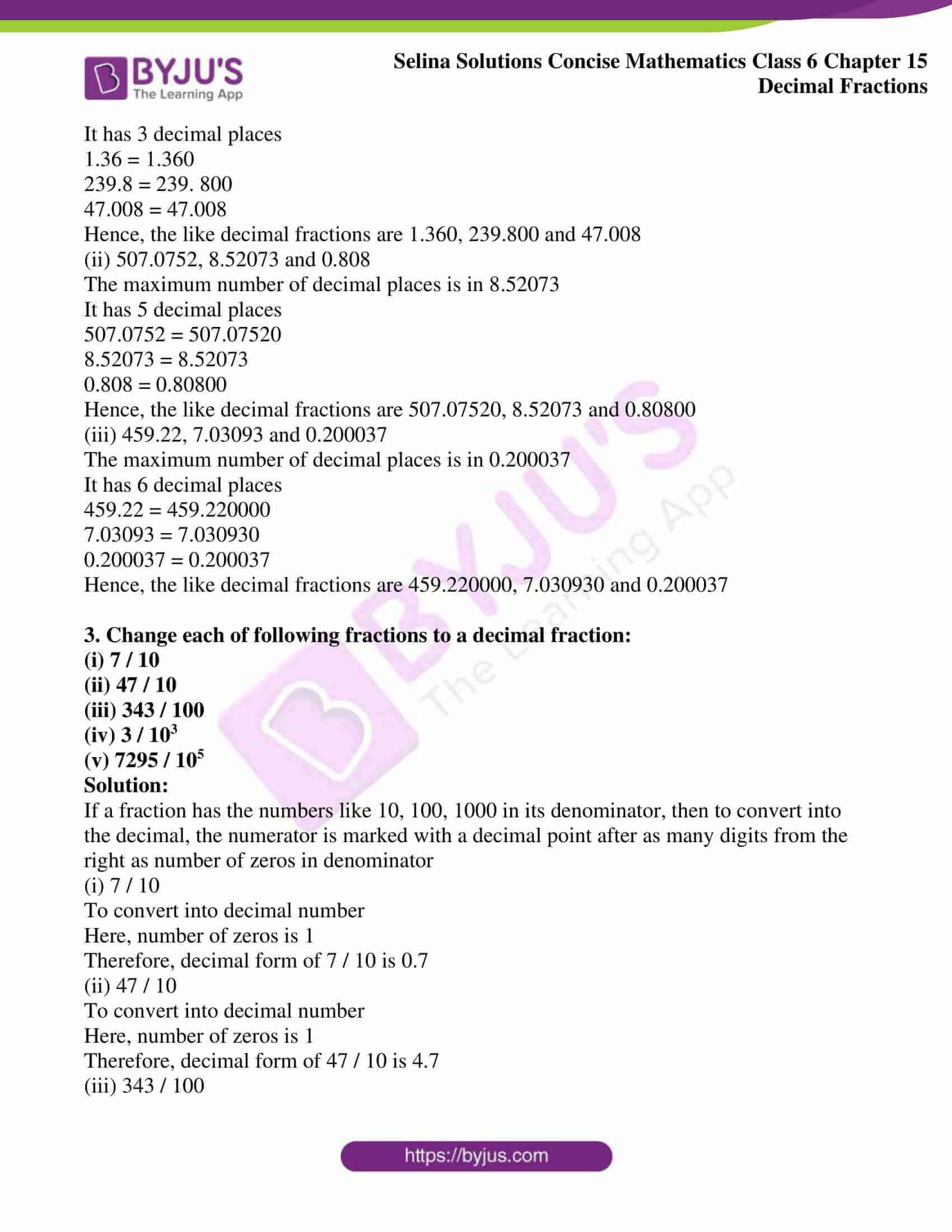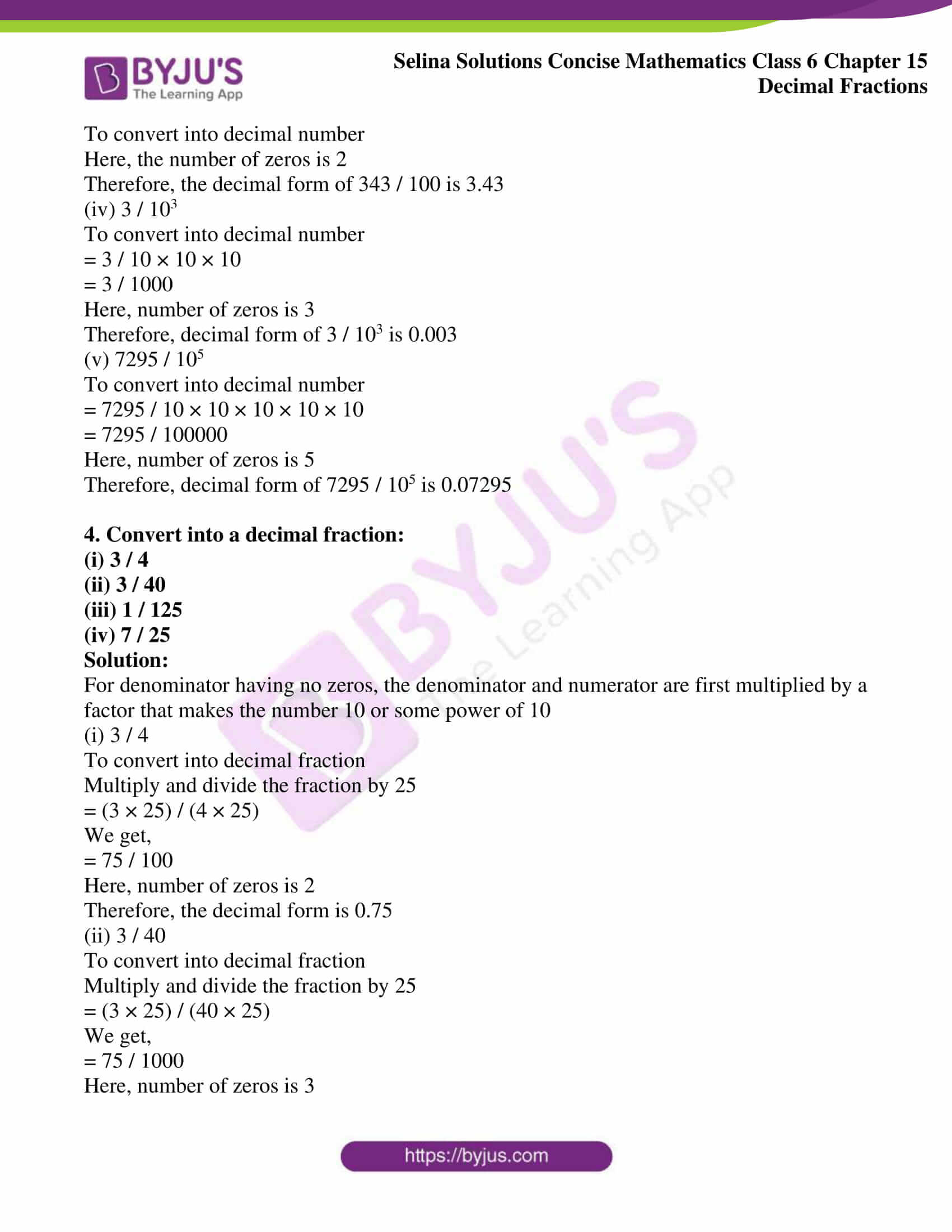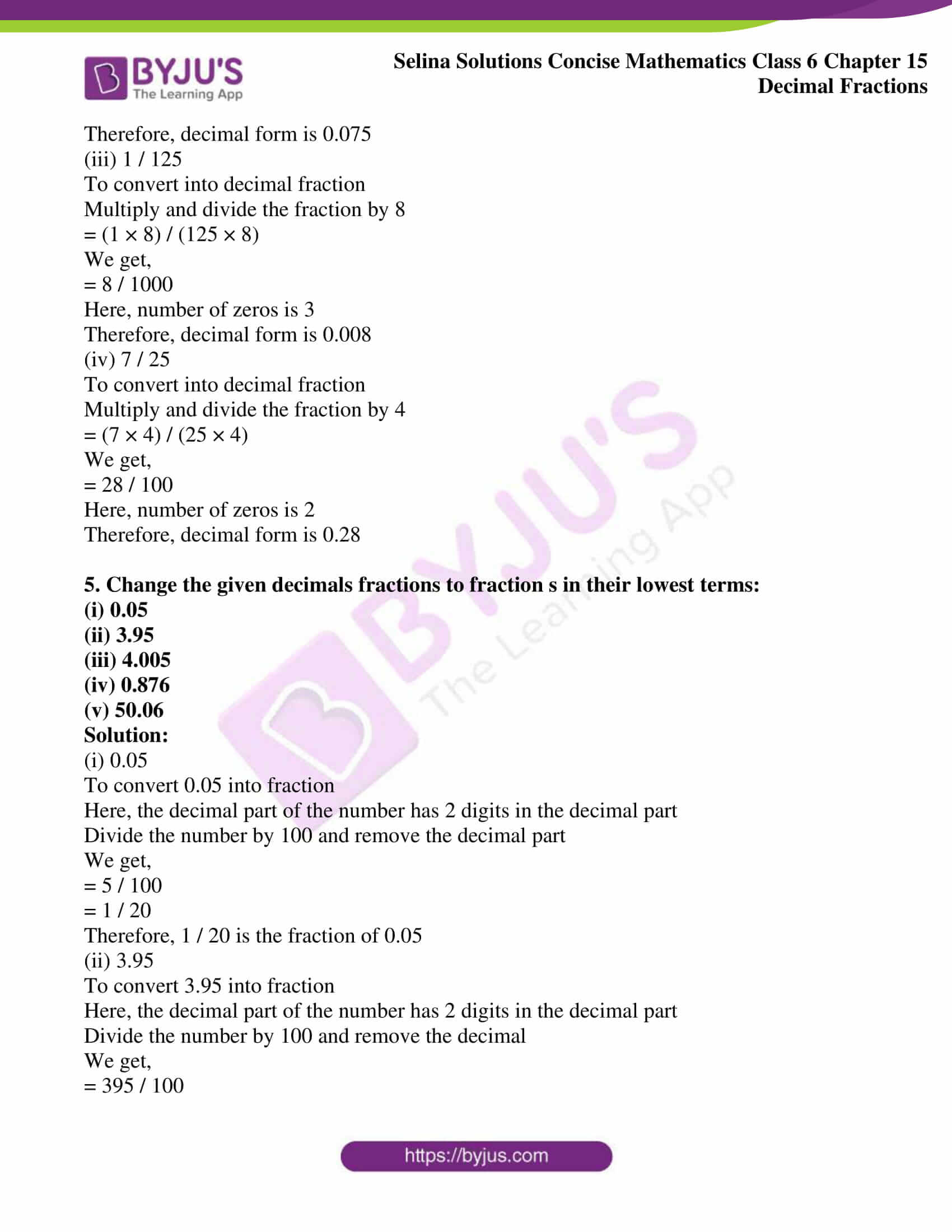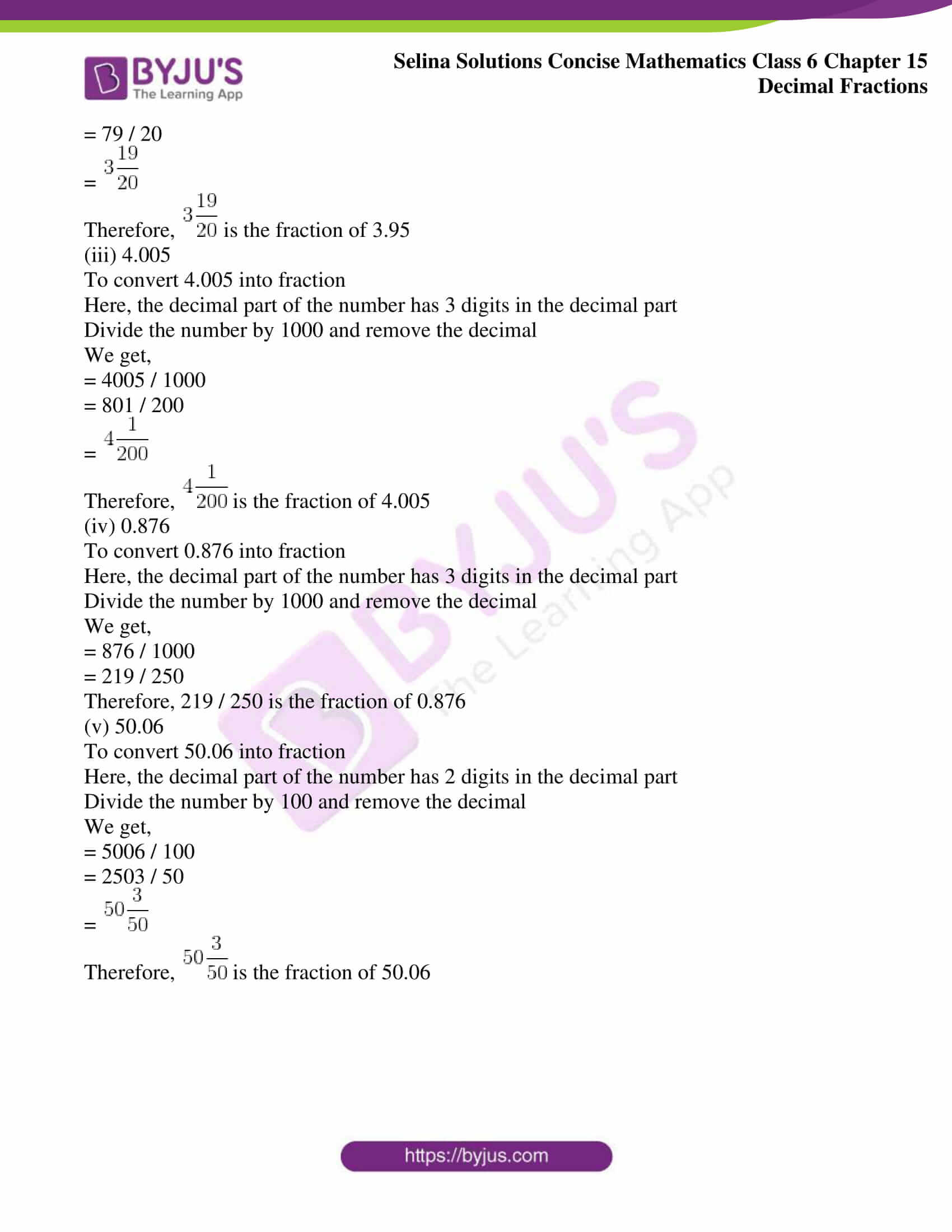### Access other exercises of Selina Solutions Concise Mathematics Class 6 Chapter 15: Decimal Fractions

Exercise 15(B) Solutions

Exercise 15(C) Solutions

Exercise 15(D) Solutions

Exercise 15(E) Solutions

### Access Selina Solutions Concise Mathematics Class 6 Chapter 15: Decimal Fractions Exercise 15(A)

Exercise 15(A)

1. Write the number of decimal places in each of the following:

(i) 7.03

(ii) 0.509

(iii) 146.2

(iv) 0.0065

(v) 8.03207

Solution:

The number of decimal places in a number is the count of digits in its decimal part.

(i) 7.03

The decimal part of the number 7.03 is .03

7.03 has 2 digits in the decimal part

Therefore, 7.03 has 2 decimal places

(ii) 0.509

The decimal part of the number 0.509 is .509

0.509 has 3 digits in the decimal part

Therefore, 0.509 has 3 decimal places

(iii) 146.2

The decimal part of the number 146.2 is .2

146.2 has 1 digit in the decimal part

Therefore, 146.2 has 1 decimal place

(iv) 0.0065

The decimal part of the number 0.0065 is .0065

0.0065 has 4 digits in the decimal part

Therefore, 0.0065 has 4 decimal places

(v) 8.03207

The decimal part of the number 8.03207 is .03207

8.03207 has 5 digits in the decimal part

Therefore, 8.03207 has 5 decimal places

2. Convert the given unlike decimal fractions into like decimal fractions:

(i) 1.36, 239.8 and 47.008

(ii) 507.0752, 8.52073 and 0.808

(iii) 459.22, 7.03093 and 0.200037

Solution:

The like decimal fractions are the numbers that have same number of decimal places. The numbers of decimal places are made equal by adding zeros in the end of number.

(i) 1.36, 239.8 and 47.008

The maximum number of decimal places is in 47.008

It has 3 decimal places

1.36 = 1.360

239.8 = 239. 800

47.008 = 47.008

Hence, the like decimal fractions are 1.360, 239.800 and 47.008

(ii) 507.0752, 8.52073 and 0.808

The maximum number of decimal places is in 8.52073

It has 5 decimal places

507.0752 = 507.07520

8.52073 = 8.52073

0.808 = 0.80800

Hence, the like decimal fractions are 507.07520, 8.52073 and 0.80800

(iii) 459.22, 7.03093 and 0.200037

The maximum number of decimal places is in 0.200037

It has 6 decimal places

459.22 = 459.220000

7.03093 = 7.030930

0.200037 = 0.200037

Hence, the like decimal fractions are 459.220000, 7.030930 and 0.200037

3. Change each of following fractions to a decimal fraction:

(i) 7 / 10

(ii) 47 / 10

(iii) 343 / 100

(iv) 3 / 103

(v) 7295 / 105

Solution:

If a fraction has the numbers like 10, 100, 1000 in its denominator, then to convert into the decimal, the numerator is marked with a decimal point after as many digits from the right as number of zeros in denominator

(i) 7 / 10

To convert into decimal number

Here, number of zeros is 1

Therefore, decimal form of 7 / 10 is 0.7

(ii) 47 / 10

To convert into decimal number

Here, number of zeros is 1

Therefore, decimal form of 47 / 10 is 4.7

(iii) 343 / 100

To convert into decimal number

Here, the number of zeros is 2

Therefore, the decimal form of 343 / 100 is 3.43

(iv) 3 / 103

To convert into decimal number

= 3 / 10 × 10 × 10

= 3 / 1000

Here, number of zeros is 3

Therefore, decimal form of 3 / 103 is 0.003

(v) 7295 / 105

To convert into decimal number

= 7295 / 10 × 10 × 10 × 10 × 10

= 7295 / 100000

Here, number of zeros is 5

Therefore, decimal form of 7295 / 105 is 0.07295

4. Convert into a decimal fraction:

(i) 3 / 4

(ii) 3 / 40

(iii) 1 / 125

(iv) 7 / 25

Solution:

For denominator having no zeros, the denominator and numerator are first multiplied by a factor that makes the number 10 or some power of 10

(i) 3 / 4

To convert into decimal fraction

Multiply and divide the fraction by 25

= (3 × 25) / (4 × 25)

We get,

= 75 / 100

Here, number of zeros is 2

Therefore, the decimal form is 0.75

(ii) 3 / 40

To convert into decimal fraction

Multiply and divide the fraction by 25

= (3 × 25) / (40 × 25)

We get,

= 75 / 1000

Here, number of zeros is 3

Therefore, decimal form is 0.075

(iii) 1 / 125

To convert into decimal fraction

Multiply and divide the fraction by 8

= (1 × 8) / (125 × 8)

We get,

= 8 / 1000

Here, number of zeros is 3

Therefore, decimal form is 0.008

(iv) 7 / 25

To convert into decimal fraction

Multiply and divide the fraction by 4

= (7 × 4) / (25 × 4)

We get,

= 28 / 100

Here, number of zeros is 2

Therefore, decimal form is 0.28

5. Change the given decimals fractions to fraction s in their lowest terms:

(i) 0.05

(ii) 3.95

(iii) 4.005

(iv) 0.876

(v) 50.06

Solution:

(i) 0.05

To convert 0.05 into fraction

Here, the decimal part of the number has 2 digits in the decimal part

Divide the number by 100 and remove the decimal part

We get,

= 5 / 100

= 1 / 20

Therefore, 1 / 20 is the fraction of 0.05

(ii) 3.95

To convert 3.95 into fraction

Here, the decimal part of the number has 2 digits in the decimal part

Divide the number by 100 and remove the decimal

We get,

= 395 / 100

= 79 / 20

=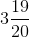Therefore,is the fraction of 3.95

(iii) 4.005

To convert 4.005 into fraction

Here, the decimal part of the number has 3 digits in the decimal part

Divide the number by 1000 and remove the decimal

We get,

= 4005 / 1000

= 801 / 200

=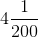Therefore,is the fraction of 4.005

(iv) 0.876

To convert 0.876 into fraction

Here, the decimal part of the number has 3 digits in the decimal part

Divide the number by 1000 and remove the decimal

We get,

= 876 / 1000

= 219 / 250

Therefore, 219 / 250 is the fraction of 0.876

(v) 50.06

To convert 50.06 into fraction

Here, the decimal part of the number has 2 digits in the decimal part

Divide the number by 100 and remove the decimal

We get,

= 5006 / 100

= 2503 / 50

=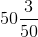Therefore,is the fraction of 50.06